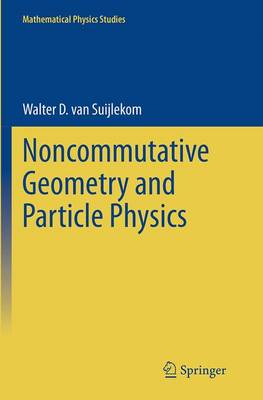•# Noncommutative Geometry and Particle Physics - Mathematical Physics Studies (Paperback)

(author)
£44.99
Paperback 237 Pages / Published: 27/09/2016
• We can order this

Usually dispatched within 3 weeks

This book provides an introduction to noncommutative geometry and presents a number of its recent applications to particle physics. It is intended for graduate students in mathematics/theoretical physics who are new to the field of noncommutative geometry, as well as for researchers in mathematics/theoretical physics with an interest in the physical applications of noncommutative geometry. In the first part, we introduce the main concepts and techniques by studying finite noncommutative spaces, providing a "light" approach to noncommutative geometry. We then proceed with the general framework by defining and analyzing noncommutative spin manifolds and deriving some main results on them, such as the local index formula. In the second part, we show how noncommutative spin manifolds naturally give rise to gauge theories, applying this principle to specific examples. We subsequently geometrically derive abelian and non-abelian Yang-Mills gauge theories, and eventually the full Standard Model of particle physics, and conclude by explaining how noncommutative geometry might indicate how to proceed beyond the Standard Model.

Publisher: Springer
ISBN: 9789402401714
Number of pages: 237
Weight: 3927 g
Dimensions: 235 x 155 x 14 mm
Edition: Softcover reprint of the original 1st ed. 201